Download PresentationShort-Term Load Forecasting Using System-Type Neural Network Architecture

# Short-Term Load Forecasting Using System-Type Neural Network Architecture - PowerPoint PPT Presentation

Short-Term Load Forecasting Using System-Type Neural Network Architecture. Shu Du, Graduate Student Mentor: Kwang Y. Lee, Professor and Chair Department of Electrical and Computer Engineering Baylor University. Outline. Introduction and Background Objectives Load Forecasting CategoriesI am the owner, or an agent authorized to act on behalf of the owner, of the copyrighted work described.
Download Presentation## Short-Term Load Forecasting Using System-Type Neural Network Architecture

An Image/Link below is provided (as is) to download presentation

Download Policy: Content on the Website is provided to you AS IS for your information and personal use and may not be sold / licensed / shared on other websites without getting consent from its author.While downloading, if for some reason you are not able to download a presentation, the publisher may have deleted the file from their server.

- - - - - - - - - - - - - - - - - - - - - - - - - - E N D - - - - - - - - - - - - - - - - - - - - - - - - - -
Presentation Transcript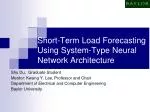### Short-Term Load Forecasting Using System-Type Neural Network Architecture

Shu Du, Graduate Student

Mentor: Kwang Y. Lee, Professor and Chair

Department of Electrical and Computer Engineering

Baylor UniversityOutline
• Introduction and Background
• Objectives
• Load Forecasting Categories
• Load Forecasting Methods
• Proposed Approach
• Regression and Rearrangement
• System-Type Neural Network Method
• Learning Algorithm of System-Type Neural Network
• Extrapolation and Interpolation
• Simulation Results
• Rearrangement
• Output of Semigroup Channel
• Extrapolation
• Conclusions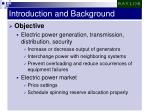Introduction and Background
• Objective
• Electric power generation, transmission, distribution, security
• Increase or decrease output of generators
• Interchange power with neighboring systems
• Prevent overloading and reduce occurrences of equipment failures
• Electric power market
• Price settings
• Schedule spinning reserve allocation properly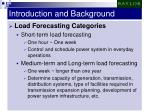Introduction and Background
• Load Forecasting Categories
• Short-term load forecasting
• One hour ~ One week
• Control and schedule power system in everyday operations
• Medium-term and Long-term load forecasting
• One week ~ longer than one year
• Determine capacity of generation, transmission, distribution systems, type of facilities required in transmission expansion planning, development of power system infrastructure, etc.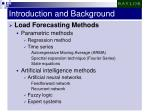Introduction and Background
• Load Forecasting Methods
• Parametric methods
• Regression method
• Time series

Autoregressive Moving Average (ARMA)

Spectral expansion technique (Fourier Series)

State equations

• Artificial intelligence methods
• Artificial neural networks

Feedforward network

Recurrent network

• Fuzzy logic
• Expert systemsProposed Approach
• Regression and Rearrangement
• Regression
• Objective

Represent given load with respect to two major variables—time and temperature

-----Base load component (time factor)

-----Weather sensitive load component (weather factor)

-----Load component (other factors)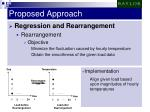Day

Temperature

Rearrangement

Hour

Hour

1

2

24

1

2

24

Proposed Approach
• Regression and Rearrangement
• Rearrangement
• Objective

Minimize the fluctuation caused by hourly temperature

Obtain the smoothness of the given load data

• Implementation

Align given load based upon magnitudes of hourly temperatures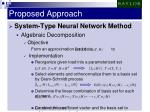Proposed Approach
• System-Type Neural Network Method
• Algebraic Decomposition
• Objective

Form an approximation load data to

• Implementation
• Reorganize given load into a parameterized set
• Select elements and orthonormalize them to a basis set by Gram-Schmidt process
• Determine the linear combination of basis set for each element
• Combine the coefficient vector and the basis set to achieve an approximation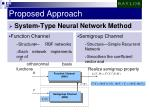Function Channel

(NN1)

Semigroup Channel

(NN2)

Proposed Approach
• System-Type Neural Network Method
• Function Channel
• Structure— RBF networks
• Each network implements one of

orthonormal basis functions

• Semigroup Channel
• Structure—Simple Recurrent Network
• Smoothen the coefficient vector and

Realize semigroup property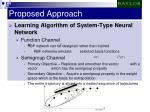Proposed Approach
• Learning Algorithm of System-Type Neural Network
• Function Channel
• RBF network can be designed rather than trained
• RBF networks emulate selected basis functions
• Semigroup Channel
• Primary Objective – Replicate and smoothen the vector with a vector which has the semigroup property
• Secondary Objective – Acquire a semigroup property in the weight space which is the basis for extrapolation
• The entire trajectory is sliced into a nested sequence of trajectoriesTemperature

Temperature

Extrapolated Coefficient

Interpolated Coefficient

4

4

Decompose & Smoothen

Decompose & Smoothen

3

3

2

2

1

1

Hour

Hour

4

3

4

5

1

1

2

2

24

24

Proposed Approach
• Extrapolation and Interpolation
• Extrapolation
• Extrapolation is needed only when temperature forecast at a given hour exceeds the historical bounds at the same time
• Interpolation
• Interpolation is needed when temperature forecast at a given hour falls into the historical temperature range at the same time

Extrapolation of Coefficient

Interpolation of CoefficientSimulation Results
• Forecasting Procedure
• Data Source
• New England Independent System Operator
• Historical Data
• Load – load for the year 2002
• Temperature – weighted average hourly temperature of 8 stations in

the New England area

• Pattern
• Weekday pattern (Mon ~ Fri) and Weekend pattern (Sat, Sun)
• Next Day Forecasting
• Previous loads and temperatures in the length of four weeksSimulation Results
• Simulation of Forecasting A Weekday Load
• Rearrangement

RearrangeSimulation Results
• Simulation of Forecasting A Weekday Load
• Output of Semigroup ChannelSimulation Results
• Simulation of Forecasting A Weekday Load
• ExtrapolationSimulation Results
• Regression Load Forecasting ResultsConclusions
• Next Day Load Forecasting based upon Weather Forecast
• A mathematical approach referred to as algebraic decomposition is investigated
• The system-type neural network architecture combining Radial Basis Function Networks and a Simple Recurrent Network is proposed
• A new training algorithm in the SRN is proposed
• Regression and Rearrangement are performed to guarantee smoothness of coefficient vector
• Interpolation and Extrapolation are implemented based on temperatures
• Much better results with respect to actual load and removal of regression are expected if load and temperature are highly correlated to each other